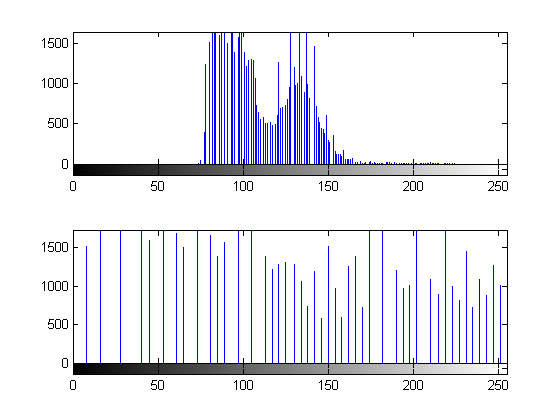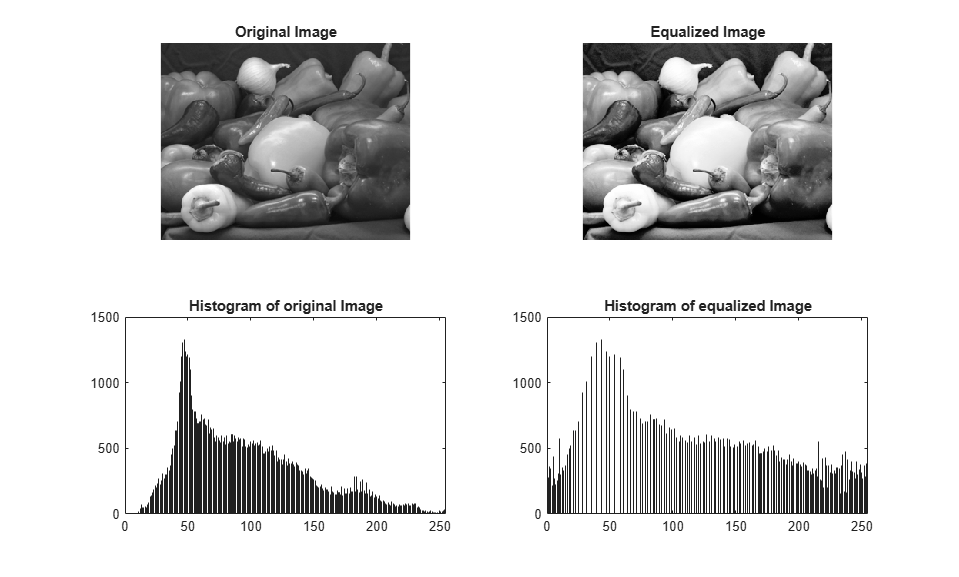# Image Enhancement by Histogram Equalization

This example shows how to generate HDL code from a MATLAB® design that does image enhancement using histogram equalization.

### Algorithm

The Histogram Equalization algorithm enhances the contrast of images by transforming the values in an intensity image so that the histogram of the output image is approximately flat.

```I = imread('pout.tif'); J = histeq(I); subplot(2,2,1); imshow( I ); subplot(2,2,2); imhist(I) subplot(2,2,3); imshow( J ); subplot(2,2,4); imhist(J) ```### MATLAB Design

```design_name = 'mlhdlc_heq'; testbench_name = 'mlhdlc_heq_tb'; ```

Let us take a look at the MATLAB design

```type(design_name); ```
```%%%%%%%%%%%%%%%%%%%%%%%%%%%%%%%%%%%%%%%%%%%%%%%%%%%%%%%%%%%%%%%%%%%%%%%%%%% % heq.m % Histogram Equalization Algorithm %%%%%%%%%%%%%%%%%%%%%%%%%%%%%%%%%%%%%%%%%%%%%%%%%%%%%%%%%%%%%%%%%%%%%%%%%%% function [x_out, y_out, pixel_out] = ... mlhdlc_heq(x_in, y_in, pixel_in, width, height) % Copyright 2011-2015 The MathWorks, Inc. persistent histogram persistent transferFunc persistent histInd persistent cumSum if isempty(histogram) histogram = zeros(1, 2^14); transferFunc = zeros(1, 2^14); histInd = 0; cumSum = 0; end % Figure out indexes based on where we are in the frame if y_in < height && x_in < width % valid pixel data histInd = pixel_in + 1; elseif y_in == height && x_in == 0 % first column of height+1 histInd = 1; elseif y_in >= height % vertical blanking period histInd = min(histInd + 1, 2^14); elseif y_in < height % horizontal blanking - do nothing histInd = 1; end %Read histogram (must be outside conditional logic) histValRead = histogram(histInd); %Read transfer function (must be outside conditional logic) transValRead = transferFunc(histInd); %If valid part of frame add one to pixel bin and keep transfer func val if y_in < height && x_in < width histValWrite = histValRead + 1; %Add pixel to bin transValWrite = transValRead; %Write back same value cumSum = 0; elseif y_in >= height %In blanking time index through all bins and reset to zero histValWrite = 0; transValWrite = cumSum + histValRead; cumSum = transValWrite; else histValWrite = histValRead; transValWrite = transValRead; end %Write histogram (must be outside conditional logic) histogram(histInd) = histValWrite; %Write transfer function (must be outside conditional logic) transferFunc(histInd) = transValWrite; pixel_out = transValRead; x_out = x_in; y_out = y_in; ```
```type(testbench_name); ```
```%Test bench for Histogram Equalization % Copyright 2011-2018 The MathWorks, Inc. testFile = 'mlhdlc_img_peppers.png'; imgOrig = imread(testFile); [height, width] = size(imgOrig); imgOut = zeros(height,width); hBlank = 20; % make sure we have enough vertical blanking to filter the histogram vBlank = ceil(2^14/(width+hBlank)); for frame = 1:2 disp(['working on frame: ', num2str(frame)]); for y_in = 0:height+vBlank-1 %disp(['frame: ', num2str(frame), ' of 2, row: ', num2str(y_in)]); for x_in = 0:width+hBlank-1 if x_in < width && y_in < height pixel_in = double(imgOrig(y_in+1, x_in+1)); else pixel_in = 0; end [x_out, y_out, pixel_out] = ... mlhdlc_heq(x_in, y_in, pixel_in, width, height); if x_out < width && y_out < height imgOut(y_out+1,x_out+1) = pixel_out; end end end % normalize image to 255 imgOut = round(255*imgOut/max(max(imgOut))); figure(1) subplot(2,2,1); imshow(imgOrig, [0,255]); title('Original Image'); subplot(2,2,2); imshow(imgOut, [0,255]); title('Equalized Image'); subplot(2,2,3); histogram(double(imgOrig(:)),2^14-1); axis([0, 255, 0, 1500]) title('Histogram of original Image'); subplot(2,2,4); histogram(double(imgOut(:)),2^14-1); axis([0, 255, 0, 1500]) title('Histogram of equalized Image'); end ```

### Simulate the Design

It is always a good practice to simulate the design with the testbench prior to code generation to make sure there are no runtime errors.

```mlhdlc_heq_tb ```
```working on frame: 1 working on frame: 2 ```### Create a New HDL Coder™ Project

```coder -hdlcoder -new mlhdlc_heq_prj ```

Next, add the file 'mlhdlc_heq.m' to the project as the MATLAB Function and 'mlhdlc_heq_tb.m' as the MATLAB Test Bench.

Refer to Get Started with MATLAB to HDL Workflow for a more complete tutorial on creating and populating MATLAB HDL Coder projects.

### Run Fixed-Point Conversion and HDL Code Generation

Launch HDL Advisor and right click on the 'Code Generation' step and choose the option 'Run to selected task' to run all the steps from the beginning through the HDL code generation.

Examine the generated HDL code by clicking on the hyperlinks in the Code Generation Log window.